# Logic gate (electronics) || logic gate formula || basic concept of gate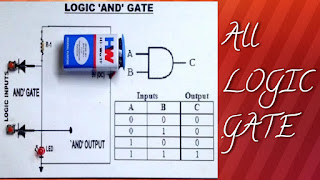## LOGIC GATE (ELECTRONICS)

We know that that a digital circuit works on two voltage level : 0 and 1.0 and 1 find linkage to the binary number system , There are two symbols or positive values , 0 and 1 . These  two number are represent all the number quantity using binary number.

The basic building block of digital circuit is a logic gate .The logic gate enter two or more input signals for designed and produced one output signals .The terminal of logic gate are two conditions 0(for low) & 1 (For high)

The basic gate are AND , OR, & NOT gate where the complex gate are NAND , NOR , & XOR gate & XNOR gate. And NAND gate and NOR gate are called universal gate.

AND gate:

The function of AND gate is identical .AND gates takes two or more signal and return only one signal . AND gate is called logic multiplication.
truth table :AND gate for 2-input

The boolean expression for the 2-input gate is F = A . B
for the 3-input gates is F= A . B . CAND gate for 2-input

OR gate:

#### The function of ORgate is identical.ORgates takes two or more signal as input and give output only one output. OR gate is called logic addition.

Truth table:OR gate for 2-input

The Boolean expression for the 2-input gate is F = A + B
for the 3-input gate is F= A + B + COR gate for 2-input

NOT gate:

The function  of NOT gate is identical to that of NOT gate operator. The NOT gate circuit inverts the logic sense of binary signals .so therefor called Inverters.
Truth table:NOT gate for 2-input

The Boolean expression for the 2-inputs gate is F = A'NOT gate for 2-input

NAND gate:

This gate is combination of AND and NOT gates. So it is a complex gate. The graphically combination of NAND gate is AND gate symbol.
Truth table: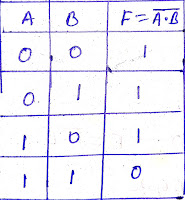NAND gate for 2-input

The Boolean expression for 2-input gate is F = (A.B)'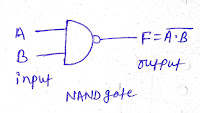NAND gate for 2-input

NOR gate:

The gate of function is combination of the OR and NOT gate . The graphically symbol of NOR gate symbol with bubble .NOR gate is also reffered to as inverted OR gate.
Truth table:NOR gate for 2-input

The Boolean expression for 2-inputs gate is  F = (A + B)'
for 3-inputs gate is  F= (A + B + C)'NOR gate for 2-input

XOR gate:

The XOR gate stand for exclusive OR gate. XOR gate are use for two way commonly use in  our homes for staircase light .
Truth table:XOR gate for 2-input

The Boolean expression for 2-input gate is F = (A+B) . (A'+B')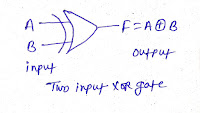XOR gate for 2-input

XNOR gate:

Truth table: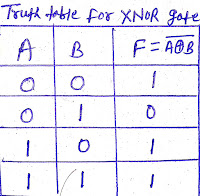XNOR gate for 2-input

The Boolean expression for 2-input XNOR gate F = A . B + A' . B'
3-input XNOR gate F = A . B . C + A' . B' . C'XNOR gate for 2 and 3 -inputs